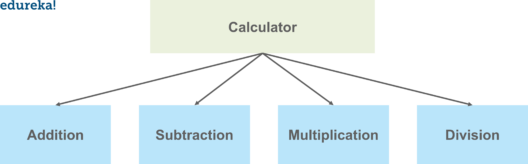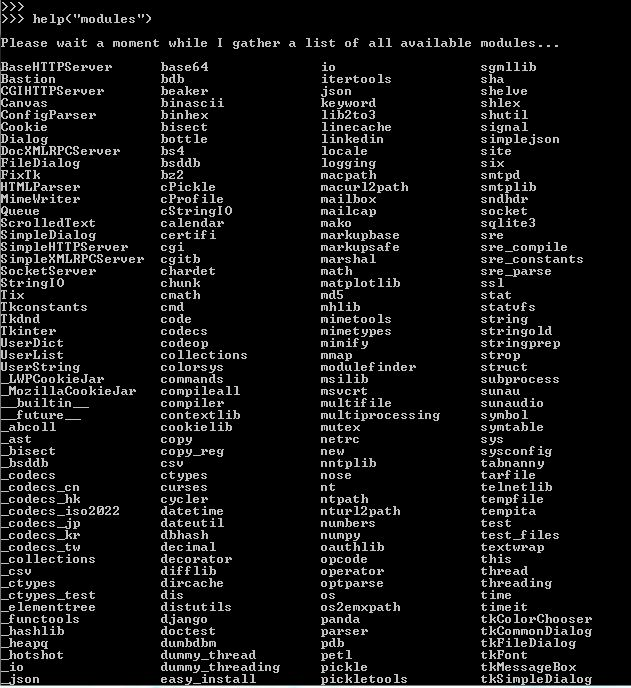This article was published as a part of the Data Science Blogathon

## Introduction

Nowadays, the Python programming language becomes one of the most popular languages. When we write the codes for Production level Data Science Projects, what happens is that our Python code grows in size, and as a result most probably it becomes unorganized over time. So, keeping your code in the same file as it grows makes your code difficult to maintain and debug.

So, to resolve these kinds of issues, Python modules help us to organize and group the content by using files and folders. This modular programming approach where we have broken the code into separate parts is where python modules come into the picture. So, In this article, I will help you to understand the complete intuition behind modules in Python in a detailed manner.

Note: If you are more interested in learning concepts in an Audio-Visual format, So to learn the basic concepts of Python Modules and some other related stuff you may see this video.

The topics which we are going to discuss in this article are as follows:

• What are Python Modules?
• How to create Python Modules?
• How to use Python Modules?
• How we can introduce variables in Python Modules?
• How to rename a Python Module?
• How does import from modules work?
• What are the advantages to use modules in Python?
• Working with Built-in Modules in Python – Math, and Statistics Module.Image Source:

So, Let’s Get Started,

## What are Python Modules?

In Python, Modules are simply files with the “.py” extension containing Python code that can be imported inside another Python Program.

In simple terms, we can consider a module to be the same as a code library or a file that contains a set of functions that you want to include in your application.

With the help of modules, we can organize related functions, classes, or any code block in the same file. So, It is considered a best practice while writing bigger codes for production-level projects in Data Science is to split the large Python code blocks into modules containing up to 300–400 lines of code.

The module contains the following components:

• Definitions and implementation of classes,
• Variables, and
• Functions that can be used inside another program.

Let’s try to gain more understanding of the concept with the help of an example:

Suppose we want to make an application for a calculator. We want to include few operations in our application such as addition, subtraction, multiplication, division, etc.

Now, here what we will be doing is to break the complete code into separate parts and simply create one module for all these operations or separate modules for each of the operations. And then we can call these modules in our main program logic.

Here the core idea is to minimize the code, and if we create modules, it doesn’t mean we can only use it for this program, but we can even call these modules for other programs as well.Image Source:

Now that we have understood the concept of modules, let us try to understand how we can create and use a module in python and also see some other functionalities related to Modules.

## How to create Python Modules?

To create a module, we have to save the code that we wish in a file with the file extension “.py”. Then, the name of the Python file becomes the name of the module.

### For Example,

In this program, a function is created with the name “welcome” and save this file with the name mymodule.py i.e. name of the file, and with the extension “.py”.

We saved the following code in a file named mymodule.py

```def welcome(name):
print("Hello, " + name +" to Analytics Vidhya")```

## How to use Python Modules?

To incorporate the module into our program, we will use the import keyword, and to get only a few or specific methods or functions from a module, we use the from keyword.

NOTE: When we are using a function from a module, then we use the following syntax:

`module_name.function_name`

Now to use the module which we have just created, we are using the import statement:

### For Example,

In this example, we will Import the module named mymodule, and then call the welcome function with a given argument:

```import mymodule
mymodule.welcome("Chirag Goyal")```

Output:

`Hello, Chirag Goyal to Analytics Vidhya`

## Variables in Python Modules

The module can contain functions, as already described, but can also contain variables of all types such as arrays, dictionaries, objects, etc.

### For Example,

Save this code in the file mymodule.py

```person1 = {
"name": "Chirag Goyal",
"age": 19,
"country": "India"
"education”: “IIT Jodhpur"
}```

### For Example,

In this example, we will Import the module named mymodule, and then try to access the person1 dictionary components:

```import mymodule
a = mymodule.person1["age"]
b = mymodule.person1["education"]
c = mymodule.person1["country"]
print(a)```

Output:

`19`

## How to rename a Python Module?

We can name the file of the module whatever you like, but we have to note that it must have the file extension “.py”.

To rename the module name, we can create an alias when you import a module, with the help of the as keyword:

### For Example,

Create an alias for mymodule with the name new_module:

```import mymodule as new_module
a = new_module.person1["age"]
b = new_module.person1["education"]
c = new_module.person1["country"]
print(a)```

Output:

`19`

## How does Import from Modules work?

If we want to choose to import only some parts from a module, then we can do this with the help of the from keyword.

### For Example,

Now, we have a module named mymodule that has one function and one dictionary:

```def welcome(name):
print("Hello, " + name +" to Analytics Vidhya")```
```person1 = {
"name": "Chirag Goyal",
"age": 19,
"country": "India"
"education”: “IIT Jodhpur"
}```

Now, Let’s try to Import only the person1 dictionary from the module named mymodule:

```from mymodule import person1
print (person1["age"])```

Output:

`19`

NOTE: Here we have to note that when we try to import using the from keyword, then do not use the module name when referring to elements in the module.

### For Example,

Use person1[“age”], not mymodule.person1[“age”]

Some of the advantages while working with modules in Python is as follows:

### Reusability

Working with modules makes the code reusable.

### Simplicity

The module focuses on a small proportion of the problem, rather than focusing on the entire problem.

### Scoping

A separate namespace is defined by a module that helps to avoid collisions between identifiers.

## Python Built-in Modules

As we know that the Python interactive shell has a number of built-in functions. As a shell start, these functions are loaded automatically and are always available, such as,

• print() and input() for I/O,
• Number conversion functions such as int(), float(), complex(),
• Data type conversions such as list(), tuple(), set(), etc.

In addition to these many built-in functions, there are also a large number of pre-defined functions available as a part of libraries bundled with Python distributions. These functions are defined in modules which are known as built-in modules.

These built-in modules are written in C language and integrated with the Python shell.

To display a list of all of the available modules in Python Programming Language, we can use the following command in the Python console:

``help('modules') ``

The output to the above code is shown below:Now, let’s discuss some of the useful and frequently used built-in modules of Python.

• Math Module
• Statistics Module

## Working with Math Module of Python

Some of the most popular mathematical functions that are defined in the math module include,

• Trigonometric functions,
• Representation functions,
• Logarithmic functions,
• Angle conversion functions, etc.

In addition, two mathematical constants- pi and e are also defined in this module.

In Mathematics, Pi is a well-known mathematical constant. Its value is 3.141592653589793.

```>>> import math
>>> math.pi
3.141592653589793```

Another well-known mathematical constant is e, which is known as Euler’s number. Its value equals 2.718281828459045.

```>>> import math
>>> math.e
2.718281828459045```

## Trigonometric Ratios

For calculating various trigonometric ratios for a given angle, the math module contains several functions. The trigonometric functions such as sin, cos, tan, etc. take the angle argument in radians. While we are used to expressing the angle in degrees. In the math module, we have two angle conversion functions that help us to convert the angle from degrees to radians and vice versa:

• degrees()

### For Example,

In this example, we will be converting the angle of 30 degrees to radians and then back again to the degree.

NOTE: π radians is equivalent to 180 degrees.

``````>>> import math
0.5235987755982988
>>> math.degrees(math.pi/6)
29.999999999999996``````

### For Example,

In this example, we will find the value of sin, cos, and tan ratios for the angle of 30 degrees which in radians is equal to 0.5235987755982988 radians.

``````>>> import math
>>> math.sin(0.5235987755982988)
0.49999999999999994
>>> math.cos(0.5235987755982988)
0.8660254037844387
>>> math.tan(0.5235987755982988)
0.5773502691896257``````

You may also try some more functions of the math module such as math.log(), math.log10(), math.pow(). math.sqrt(), math.exp(), math.ceil(), math.floor(), etc.

## Working with Statistics Module of Python

The statistics module provides functions to mathematical statistics of numeric data. Some of the popular statistical functions are defined in this module are as follows:

• Mean
• Median
• Mode
• Standard Deviation

## Mean

The mean() method returns the arithmetic mean of the numbers present in a list.

### For Example,

```>>> import statistics
>>> statistics.mean([2,5,6,9])
5.5```

## Median

The median() method returns the middle value of numeric data present in a list.

### For Example,

```>>> import statistics
>>> statistics.median([1,2,3,7,8,9])
5.0
>>> statistics.median([1,2,3,8,9])
3.0```

## Mode

The mode() method returns the most common data point present in the list.

### For Example,

```>>> import statistics
>>> statistics.mode([2,5,3,2,8,3,9,4,2,5,6])
2```

## Standard Deviation

The stdev() method returns the standard deviation on a given sample in the form of a list.

### For Example,

```>>> import statistics
>>> statistics.stdev([1,1.5,2,2.5,3,3.5,4,4.5,5])
1.3693063937629153```

NOTE: There are also other modules in Python but here we discuss only two modules to understand how the concept of modules in Python works and you can similarly use the other Python built-in modules also.

## Other Blog Posts by Me

You can also check my previous blog posts.

Previous Data Science Blog posts.

Here is my Linkedin profile in case you want to connect with me. I’ll be happy to be connected with you.

## Email

For any queries, you can mail me on Gmail.

## End Notes

I hope that you have enjoyed the article. If you like it, share it with your friends also. Something not mentioned or want to share your thoughts? Feel free to comment below And I’ll get back to you. 😉•